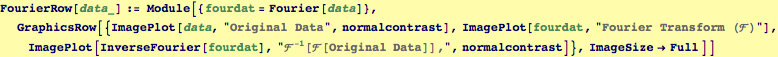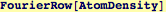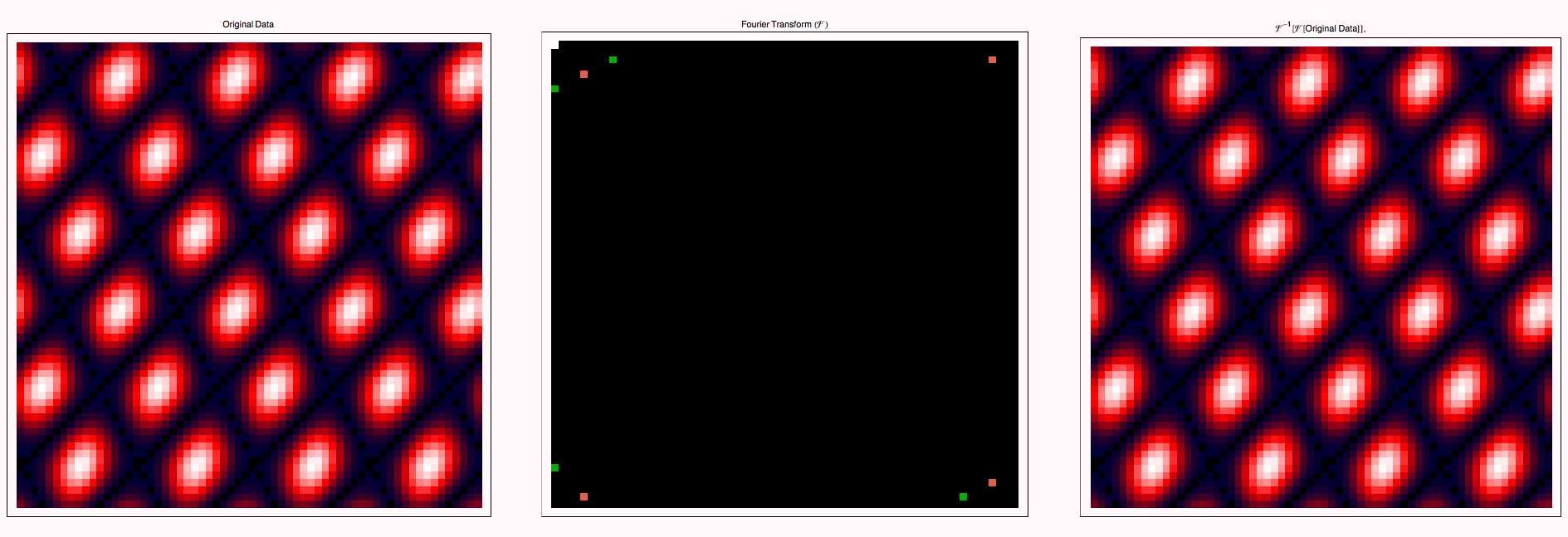Discrete Fourier Transforms on Simple 2D Lattices

We create a function that shows the original data, its Fourier transform, and then its inverse transform (hopefully) back to the original image.We will see the peaks of the image are located near (kx,ky) = 2 π (a,b)/(Image Size), where
(a,b) = {(0,0), (Image Size,0), (0,Image SIze), (Image Size, Image Size)}.  These correspond to the longest wavelength periodicities.The magnitude of fourier the fourier transform corresponds to the constructive interference of wave - like objects scattered from a real - space set of data.  When the real-space data has translational symmetry, such as in a lattice, the fourier intensity results in bright spots corresponding to periodicities present in the lattice.The distance between spots is inversely proportional to the distance between periodic scattering features, and the orientation of the (kx, ky) vector corresponds to the normal to the groups of constructively interering scatterers.

 Created by Wolfram Mathematica 6.0  (01 November 2007)